# Evaluate the probability of relapse in patients with lung cancer using artificial intelligence

This example assesses the probability of suffering relapse in lung cancer patients. We use expression data from 335 patients with eighteen thousand genes and some phenotypic variables.

This data is obtained from GEO (Gene Expression Omnibus), a public repository for functional genomics data.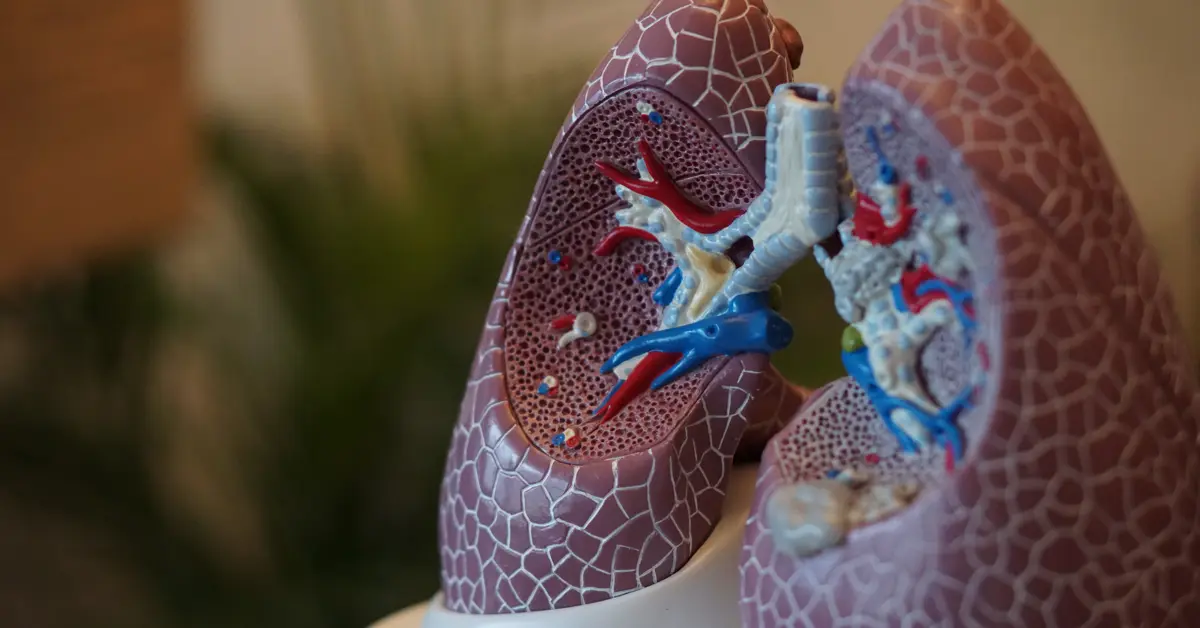### Contents:

This example is solved with Neural Designer. You can reproduce it step by step using the free trial.

## 1. Application type

The variable we will predict can have two values, "yes" if the patient has lung relapse and "no" otherwise. For that, this is a binary classification project.

Our goal is to model the probability of relapse in the lung based on gene expression and clinical data using artificial intelligence and machine learning.

## 2. Data set

The lung_cancer_relapse.csv file has the data for this example. The target variable can only be binary in a classification model: 0 (false, no) or 1 (true, yes). The number of rows (instances) in the data set is 18388, and the number of columns (variables) is 11747.

The number of input variables, or attributes for each sample, is 11745. The target variable is relapse (yes or no) wether or not the patient has suffered relapse in lung cancer. The following list summarizes the variables information:

• month: (0:60) time from the patient surgical procedure until month 60 (5 years).
• source_name: hospital source of the sample.
• sex: male or female.
• age_in_months: age of the patient in months.
• race: categories of humankind that shares certain distinctive physical traits.
• clinical_treatment_adjuvant_chemotherapy: wether or not the patient recieved chemotherapy with the surgical procedure.
• pathological_nodes: (0,1,2+) lymph node involvement relative to the TNM classification.
• pathological_tumour: (1:4) extent of the primary tumor relative to the TNM classification.
• smoking_history: patient information about their smoking habits.
• surgical_margins: margin of apparently non-tumorous tissue around a tumor that has been surgically resected.
• histologic_grade: (0, 1, 2) description of how abnormal cancer cells/tissue look under a microscope and how quickly are they likely to grow and spread.
• Genes: expression of 11734 genes from Affymetrix HG-U133A microarray normalized with RMA (Robust Multiarray Averaging) method.

To start, we use all instances. Each instance contains the input variables and the target of a patient. We have multiple instances for each patient from their surgical procedure to their month 60 (5 years) and whether or not the patient suffered a relapse in that month.

The data set is separated into training, validation, and testing. Neural Designer automatically assigns the size for each subset: 60% for training, 20% for selection, and 20% for testing.

These values can be modified at the user's choice. In our case, we will change the instance assignment from random to sequential.

Also, we can calculate the distributions for all the input variables. The following figure shows which patients had a relapse in the data set in a pie chart format.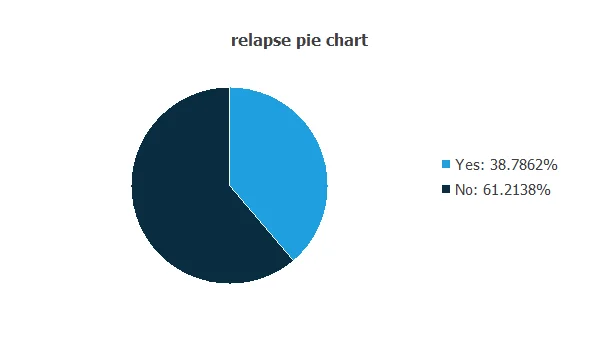The image shows that we have 61% of samples with relapse, while 39% of lung cancers without relapse.

The inputs-targets correlations might indicate to us which factors most influence our model. In this case, whether a tumor produces relapse or not and therefore be more relevant to our analysis.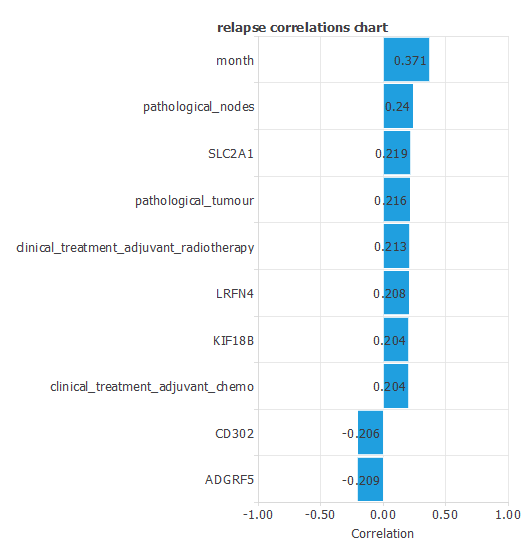The most correlated variables with the relapse variable are: month, pathological_nodes, SLC2A1 and pathological_tumour.

## 3. Neural network

The next step is to set a neural network to represent the classification function. Usually, the neural network is composed of:

The scaling layer contains the statistical values of the inputs calculated from the data file and the chosen scaling method for the input variables. Here the minimum-maximum method has been set as the scaling method. Nevertheless, the mean-standard deviation method should produce very similar results. As we use 11745 input variables, the scaling layer has 11745 inputs.

We won't use a perceptron layer to stabilize and simplify our model.

The probabilistic layer contains the method for interpreting the output values as probabilities. For our example, the probabilistic layer has 11745 inputs and one output, representing the probability of a sample relapsing. Moreover, since the activation function of the output layer is logistic, the output can already be interpreted as a probability of class membership.

The following figure represents the neural network for lung relapse estimation.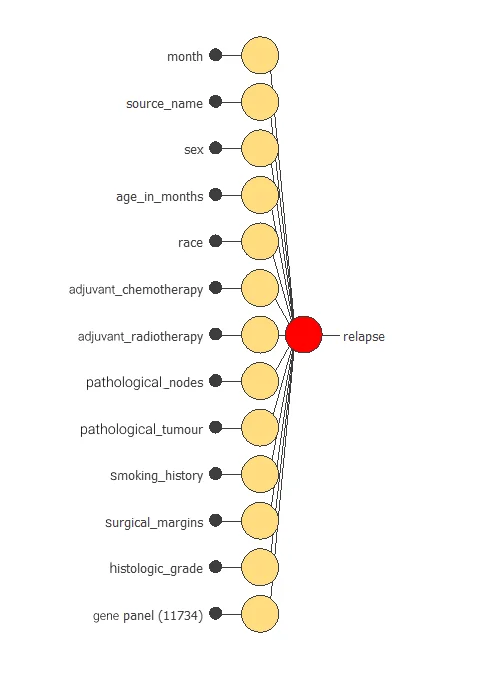As mentioned above, the network has 11745 inputs, from which we obtain a single output value. This value is the probability of lung relapse for each patient.

## 4. Training strategy

The fourth step is to set the training strategy, which is composed of two terms:

• A loss index.
• An optimization algorithm.

The loss index is the mean squared error with L2 regularization which is the default loss index for binary classification applications.

The machine learning problem is finding a neural network that minimizes the loss index. That is, a neural network that fits the data set (error term) and does not oscillate (regularization term).

The optimization algorithm that we use is the Quasi-Newton method which is also the standard optimization algorithm for this type of problem.

The following chart shows how the error decreases with the iterations during the training process. The final training and selection errors are training error = 0.03 MSE and selection error = 0.27 MSE, respectively.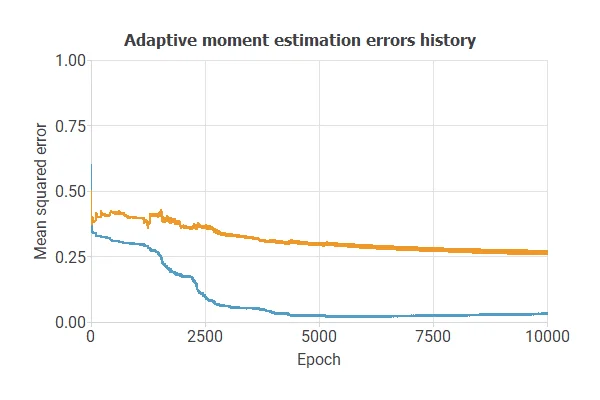As we can see in the previous image, the curves have converged. However, the selection error is greater than the training error, so we could try to continue improving the model to reduce the errors further.

## 5. Model selection

The objective of model selection is to find the network architecture which minimizes the error, that is, with the best generalization properties for the selected instances of the data set.

After performing many simulations, we have obtained an optimal model. With this model, we obtain a training error = 0.17 NSE and selection error = 0.20 NSE, respectively. Thus, we have improved our model by reducing selection error, so our model works better than before with samples not previously seen.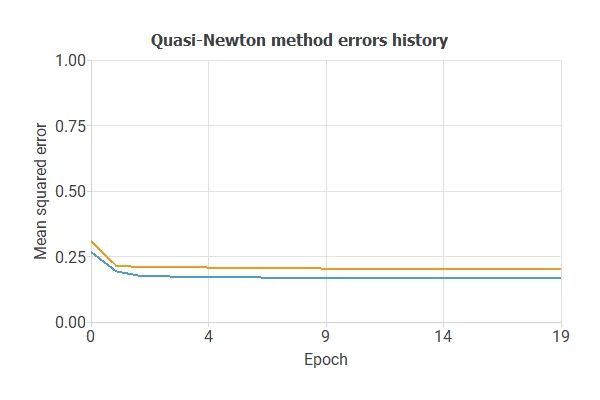Also, we have reduced the number of inputs to only 11 features. Our network is now like this:Our final network, has 11 inputs corresponding to: month, pathological_nodes, pathological_tumour, RAD51, ADGRF5, COCH, SLC2A1, CLU, ZDHHC7, LRFN4, AP2A2.

## 6. Testing analysis

The objective of the testing analysis is to validate the performance of the generalization properties of the trained neural network. To validate a classification technique, we need to compare the values provided by this technique to the observed values. We can use the ROC curve as it is the standard testing method for binary classification projects.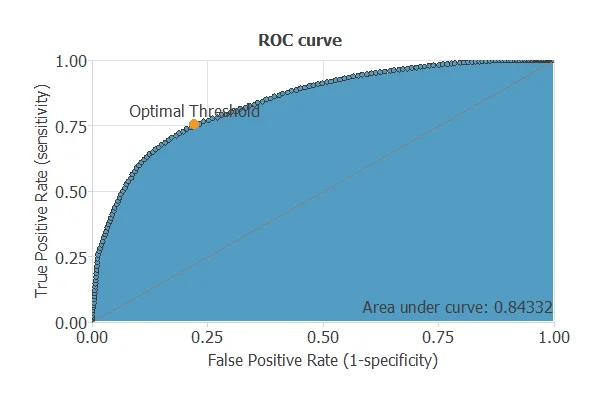A random classifier has an area under a curve of 0.5, while a perfect classifier has a value of 1. The closer this value is to 1, the better the classifier. In this example, this parameter is AUC = 0.84, which means a great performance.

The following table contains the elements of the confusion matrix. This matrix contains the true positives, false positives, false negatives, and true negatives for the variable diagnosis.

Predicted negative Predicted positive
Real negative 1222 (33.2%) 349 (9.5%)
Real positive 522 (14.2%) 1584 (43.1%)

The binary classification tests are parameters for measuring the performance of a classification problem with two classes:

• Classification accuracy (ratio of instances correctly classified): 76.31 %
• Error rate (ratio of instances misclassified): 23.68 %
• Specificity (ratio of real positive which are predicted positive): 75.21 %
• Sensitivity (ratio of real negative which are predicted negative): 77.78 %

## 7. Model deployment

Once we have tested the neural network's generalization performance, we can save it for future use in the so-called model deployment mode.

The mathematical expression represented by the neural network is written below.

```        scaled_month = month*(1+1)/(60-(0))-0*(1+1)/(60-0)-1;
scaled_pathological_nodes = pathological_nodes*(1+1)/(2-(0))-0*(1+1)/(2-0)-1;
scaled_pathological_tumour = pathological_tumour*(1+1)/(4-(1))-1*(1+1)/(4-1)-1;
scaled_COCH = COCH*(1+1)/(10.13370037-(5.095620155))-5.095620155*(1+1)/(10.13370037-5.095620155)-1;
scaled_SLC2A1 = SLC2A1*(1+1)/(9.621580124-(6.783979893))-6.783979893*(1+1)/(9.621580124-6.783979893)-1;
scaled_CLU = CLU*(1+1)/(11.18099976-(5.612390041))-5.612390041*(1+1)/(11.18099976-5.612390041)-1;
scaled_ZDHHC7 = ZDHHC7*(1+1)/(10.95040035-(8.138600349))-8.138600349*(1+1)/(10.95040035-8.138600349)-1;
scaled_LRFN4 = LRFN4*(1+1)/(9.275509834-(6.036220074))-6.036220074*(1+1)/(9.275509834-6.036220074)-1;
scaled_AP2A2 = AP2A2*(1+1)/(10.11260033-(7.864580154))-7.864580154*(1+1)/(10.11260033-7.864580154)-1;

probabilistic_layer_combinations_0 = 0.331656 +1.19441*scaled_month +0.522528*scaled_pathological_nodes +0.562851*scaled_pathological_tumour +0.106792*scaled_RAD51 -0.158342*scaled_ADGRF5 +0.0940378*scaled_COCH +0.269565*scaled_SLC2A1 -0.210056*scaled_CLU -0.244632*scaled_ZDHHC7 +0.315449*scaled_LRFN4 -0.271034*scaled_AP2A2

relapse = 1.0/(1.0 + exp(-probabilistic_layer_combinations_0);
```

The above expression can be exported, for instance, to a medical diagnosis software. It can even be embebed into a website:

Keep in mind that it is impossible to predict the future with certainty, and a physician must always interpret these predictions to make a diagnosis.